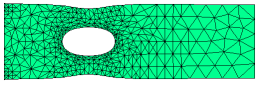﻿ Sample Problems > Applications > Stress > tension

tension

Navigation:  Sample Problems > Applications > Stress >

tension{ TENSION.PDE

This example shows the deformation of a tension bar with a hole.

The equations of Stress/Strain arise from the balance of forces in a

material medium, expressed as

dx(Sx) + dy(Txy) + Fx = 0

dx(Txy) + dy(Sy) + Fy = 0

where Sx and Sy are the stresses in the x- and y- directions,

Txy is the shear stress, and

Fx and Fy are the body forces in the x- and y- directions.

The deformation of the material is described by the displacements,

U and V, from which the strains are defined as

ex = dx(U)

ey = dy(V)

gxy = dy(U) + dx(V).

The eight quantities U,V,ex,ey,gxy,Sx,Sy and Txy are related through the

constitutive relations of the material. In general,

Sx  =  C11*ex + C12*ey + C13*gxy - b*Temp

Sy  =  C12*ex + C22*ey + C23*gxy - b*Temp

Txy =  C13*ex + C23*ey + C33*gxy

In orthotropic solids, we may take C13 = C23 = 0.

Combining all these relations, we get the displacement equations:

dx[C11*dx(U)+C12*dy(V)] + dy[C33*(dy(U)+dx(V))] + Fx = dx(b*Temp)

dy[C12*dx(U)+C22*dy(V)] + dx[C33*(dy(U)+dx(V))] + Fy = dy(b*Temp)

In the "Plane-Stress" approximation, appropriate for a flat, thin plate

loaded by surface tractions and body forces IN THE PLANE of the plate,

we may write

C11 = G         C12 = G*nu

C22 = G

C33 = G*(1-nu)/2

where G = E/(1-nu^2)

E is Young's Modulus

and   nu is Poisson's Ratio.

The displacement form of the stress equations (for uniform temperature

and no body forces) is then (dividing out G):

dx[dx(U)+nu*dy(V)] + 0.5*(1-nu)*dy[dy(U)+dx(V)] = 0

dy[nu*dx(U)+dy(V)] + 0.5*(1-nu)*dx[dy(U)+dx(V)] = 0

In order to quantify the load boundary condition mechanism,

consider the stress equations in their original form:

dx(Sx) + dy(Txy) = 0

dx(Txy) + dy(Sy) = 0

These can be written as

div(P) = 0

div(Q) = 0

where P = [Sx,Txy]

and   Q = [Txy,Sy]

The "load" (or "natural") boundary condition for the U-equation defines

the outward surface-normal component of P, while the load boundary condition

for the V-equation defines the surface-normal component of Q. Thus, the

load boundary conditions for the U- and V- equations together define

On a free boundary, both of these vectors are zero, so a free boundary

is simply specified by

Here we consider a tension strip with a hole, subject to an X-load.

}

title 'Plane Stress tension strip with a hole'

select

errlim = 1e-4       { increase accuracy to resolve stresses }

painted             { paint all contour plots }

variables

U                   { declare U and V to be the system variables }

V

definitions

nu = 0.3           { define Poisson's Ratio }

E  = 21             { Young's Modulus x 10^-11 }

 G  = E/(1-nu^2)    C11 = G    C12 = G*nu    C22 = G    C33 = G*(1-nu)/2    p1 = (1-nu)/2       initial values    U = 1    V = 1equations               { define the Plane-Stress displacement equations }

U:  dx(dx(U) + nu*dy(V)) + p1*dy(dy(U) + dx(V))  = 0

V:  dy(dy(V) + nu*dx(U)) + p1*dx(dy(U) + dx(V))  = 0

boundaries

region 1

start (0,0)

load(U)=0         { free boundary, no normal stress }

line to (3,0)     { walk bottom }

load(U)=0.1       { define an X-stress of 0.1 unit on right edge}

line to (3,1)

load(U)=0         { free boundary top }

line to (0,1)

value(U)=0       { fixed displacement on left edge }

value(V)=0

line to close

{ Cut out a hole }

start(1,0.25)

arc(center=1,0.5) angle=-360

monitors

grid(x+U,y+V)     { show deformed grid as solution progresses }

plots                 { hardcopy at to close: }

grid(x+U,y+V)     { show final deformed grid }

vector(U,V) as "Displacement"       { show displacement field }

contour(U) as "X-Displacement"

contour(V) as "Y-Displacement"

contour((C11*dx(U) + C12*dy(V))) as "X-Stress"

contour((C12*dx(U) + C22*dy(V))) as "Y-Stress"

surface((C11*dx(U) + C12*dy(V))) as "X-Stress"

surface((C12*dx(U) + C22*dy(V))) as "Y-Stress"

end